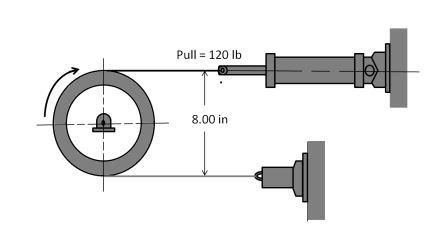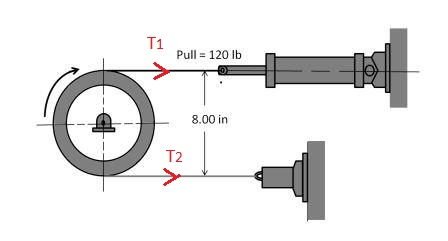# The band brake shown in Figure is actuated by a pneumatic cylinder providing a force of 120 Ib....

## Question:

The band brake shown in Figure is actuated by a pneumatic cylinder providing a force of 120 Ib. Determine the braking torque on the drum if {eq}\mu _{k} {/eq} = 0.40.## Band Brake Equation:

Due to a pulling force, let the tension in the tight portion of the band be {eq}T_1 {/eq} and on the slack portion be {eq}T_2 {/eq}. The band is making a wrapping angle of {eq}\theta {/eq} over the drum having a coefficient of friction as {eq}\mu. {/eq}

The relation between the two tension on the band is given by

{eq}\begin{align*} \frac{T_1}{T_2}& = e^{ \displaystyle \mu \theta } \\ \end{align*} {/eq}

## Answer and Explanation:Here we mark the tension on the band as {eq}T_1 \ and \ T_2. {/eq}

• The tension {eq}T_1 =120 \ lb {/eq} as actuated by the pneumatic cylinder.
• The coefficient of friction on the drum is {eq}\mu _{k} {/eq} = 0.40.
• Angle of wrapping of the band over the drum is {eq}\theta = \pi {/eq}.
• Radius of the drum is r = (8.00 / 2) in = 4.00 in.

From the band brake equation we have

{eq}\begin{align*} \frac{T_1}{T_2}& = e^{ \displaystyle \mu _{k} \theta } \\ \frac{120 \ lb}{T_2}& = e^{\displaystyle 0.4 \times \pi } \\ T_2 & = 34.17 \ lb \end{align*} {/eq}

The braking torque is given by

{eq}\begin{align*} \tau & = (T_1-T_2) \cdot r \\ & = (120-34.17) \cdot 4.00 \ lb.in \\ & = 343.3 \ lb.in (Answer) \end{align*} {/eq}

#### Learn more about this topic:Torque: Concept, Equation & Example

from UExcel Physics: Study Guide & Test Prep

Chapter 7 / Lesson 4
19K